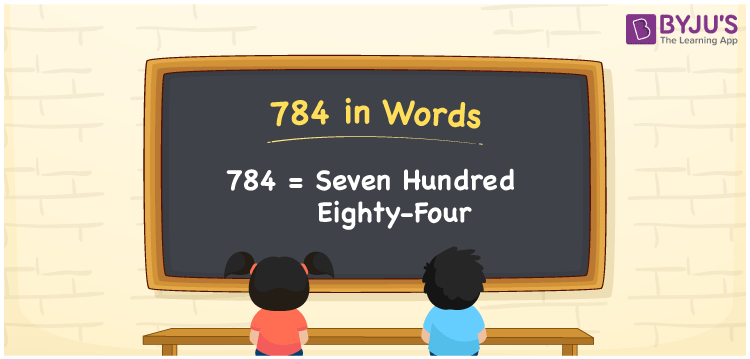# 784 in Words

784 in words is written as Seven hundred eighty-four. In both the International System of Numerals and the Indian System of Numerals, 784 is written as Seven hundred eighty-four. The number 784 is a Cardinal Number as it represents some quantity. For example, “cost of the dress is rupees 784”.

 784 in Words Seven hundred eighty-four Seven hundred eighty-four in Number 784

## 784 in English Words

We write 784 in English Words using the letters of the English alphabet. Therefore, we read 784 in English as “Seven hundred eighty-four”.## How to Write 784 in Words?

To write 784 in words, we shall use the place value chart. In the place value chart, write 7 in the hundreds, 8 in the tens, and 4 in the ones, respectively. Now let us make a place value chart to write the number 784 in words.

 Hundreds Tens Ones 7 8 4

Thus, we can write the expanded form as

7 × Hundred + 8 × Ten + 4 × One

= 7 × 100 + 8 × 10 + 4 × 1

= 700 + 80 + 4

= 784

= Seven hundred eighty-four.

784 is a natural number, the successor of 783 and the predecessor of 785.

784 in words – Seven hundred eighty-four

• Is 784 an odd number? – No
• Is 784 an even number? – Yes
• Is 784 a perfect square number? – Yes; 28 × 28 = 784
• Is 784 a perfect cube number? – No
• Is 784 a prime number? – No
• Is 784 a composite number? – Yes

## Frequently Asked Questions on 784 in Words

Q1

### How to write 784 in words?

784 in words is written as Seven hundred eighty-four.
Q2

### How to write 784 in the International and Indian System of Numerals?

In both, the system of numerals, 784 in words, is written as Seven hundred eighty-four.
Q3

### What is the successor of 784?

The successor of 784 is 785.